# Avoid collision using linear probing

#### Collision

While hashing, two or more key points to the same hash index under some modulo M is called as collision.

In this tutorial, we will learn how to avoid collison using linear probing technique.

## Linear Probing

Calculate the hash key. key = data % size;

If hashTable[key] is empty, store the value directly. hashTable[key] = data.

If the hash index already has some value, check for next index.

key = (key+1) % size;

If the next index is available hashTable[key], store the value. Otherwise try for next index.

Do the above process till we find the space.

## Linear Probing Procedure

#### Initial Hash Table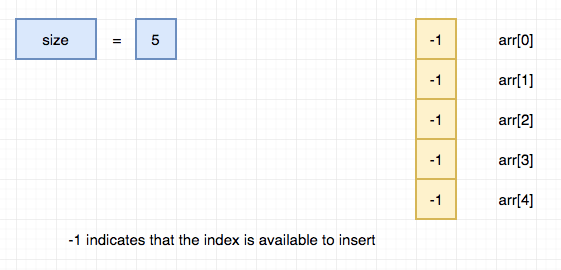#### Insert 13#### insert 1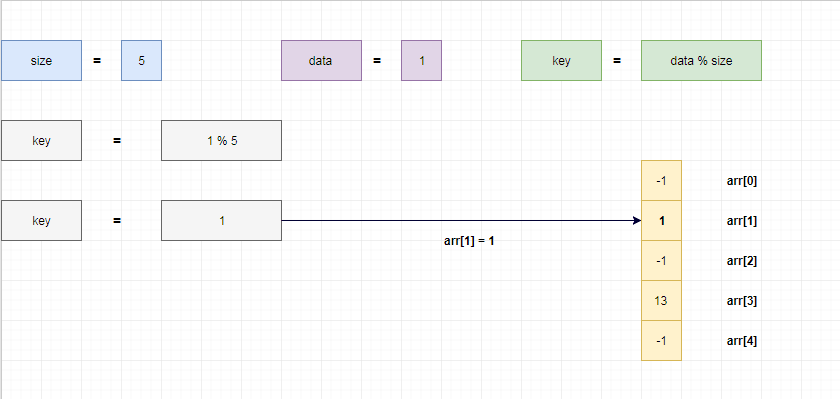#### Insert 6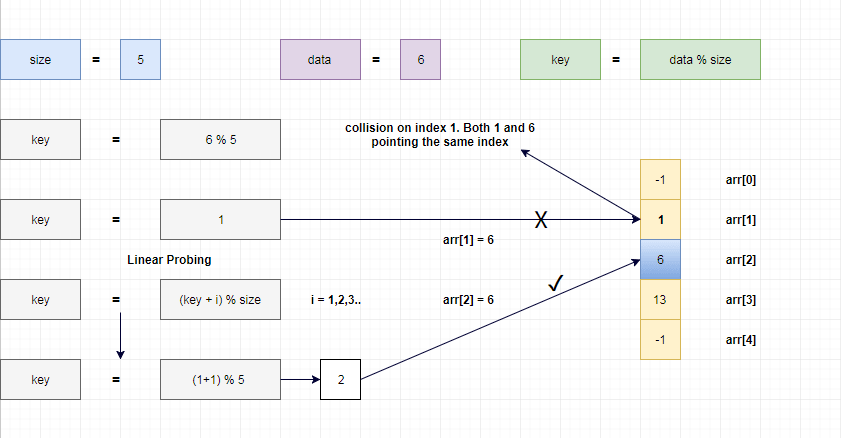1 % 5 = 1.

6 % 5 = 1.

Both 1 and 6 points the same index under modulo 5.

So that we have placed 6 in arr which is next available index.

#### Insert 11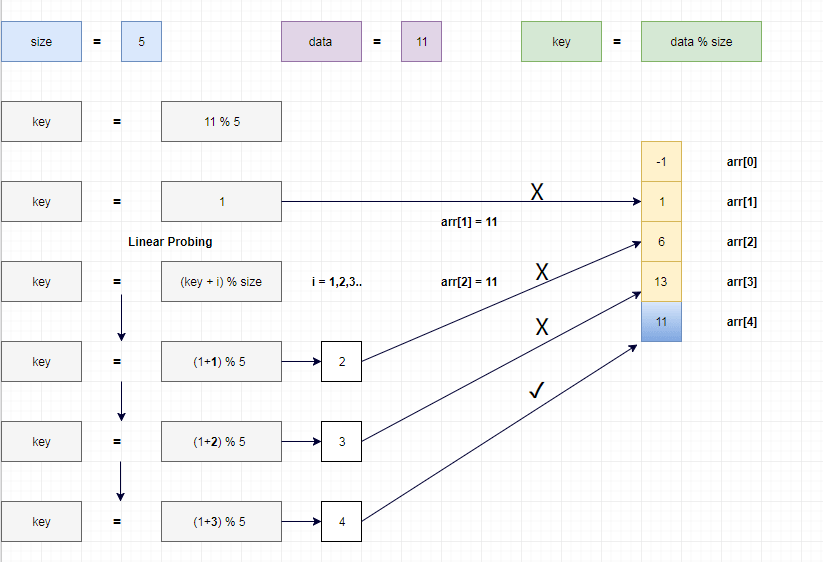1 % 5 = 1.

6 % 5 = 1.

11 % 5 = 1.

Both 1, 6 and 11 points the same index under modulo 5.

So that we have placed 11 in arr which is next available index.

#### Insert 10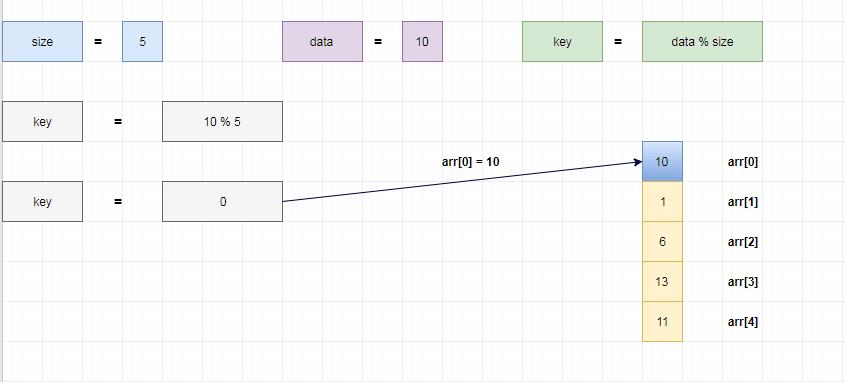#### Insert 15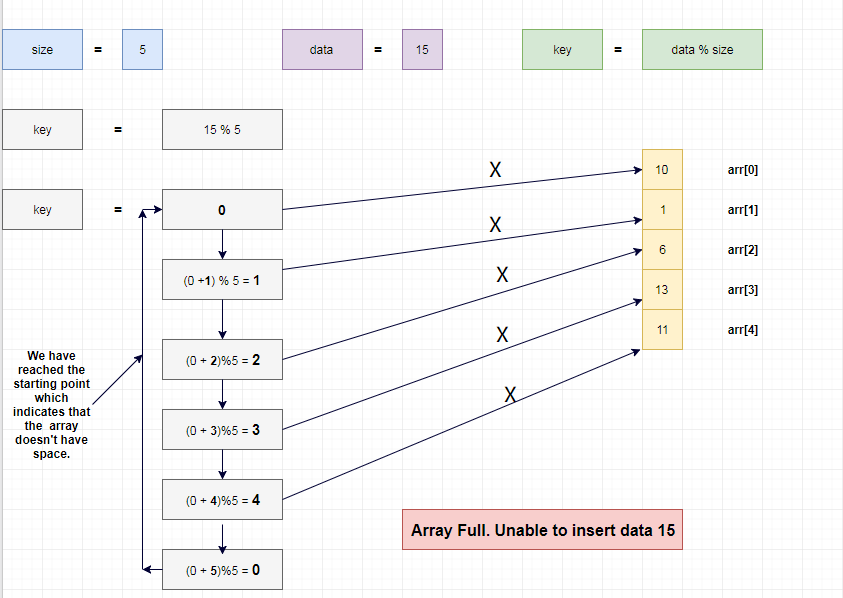15 % 5 = 0.

Hash table don't have any empty index. So, we can't insert the data.